# GSEB Solutions Class 6 Maths Chapter 13 Symmetry Ex 13.3

Gujarat Board GSEB Textbook Solutions Class 6 Maths Chapter 13 Symmetry Ex 13.3 Textbook Questions and Answers.

## Gujarat Board Textbook Solutions Class 6 Maths Chapter 13 Symmetry Ex 13.3

Question 1.
Find the number of lines of symmetry in each of the following shapes. How will you check your answers?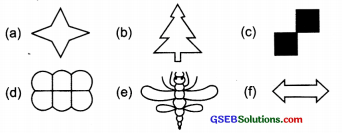Solution:
By drawing the lines of symmetry, we find that:

(a) There are four lines of symmetry.(b) There is one line of symmetry.(c) There are two lines of symmetry.(d) There are two lines of symmetry.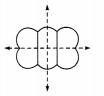(e) There is one line of symmetry.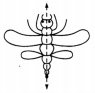(f) There are two lines of symmetry.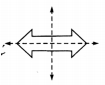Question 2.
Copy the following drawings on squared paper. Complete each one of them such that the resulting figure has two dotted lines as two lines of symmetry. How did you go about completing the picture?Solution:
Completing the given figures we have: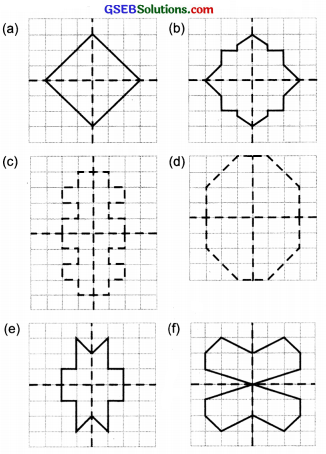Question 3.In each figure alongside, a letter of the alphabet is shown along with a vertical line. Take the mirror image of the letter in the given line. Find which letters look the same after reflection (i.e. which letters look the same in the image) and which do not. Can you guess why?
Try for O E M N P H LT S V X
Solution:
Taking the mirror image of A, we find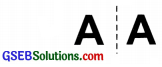That the letter A after reflection looks the same.
Whereas the mirror image of B,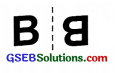does not look the same as B.
Similarly, the mirror images of the given letters are given as: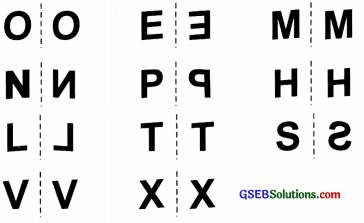Obviously, the letters A, O, M, H, T, V, and X look the same after reflection because all these letters are symmetrical. On the other hand letters B, E, N, P, L, and S do not look the same after reflection.